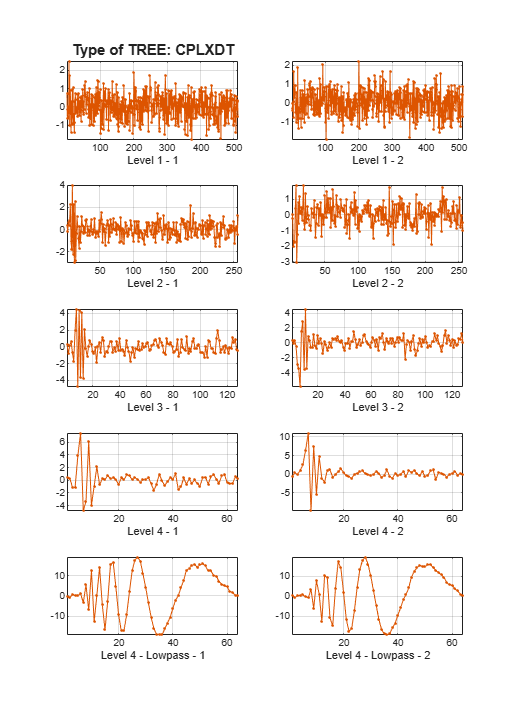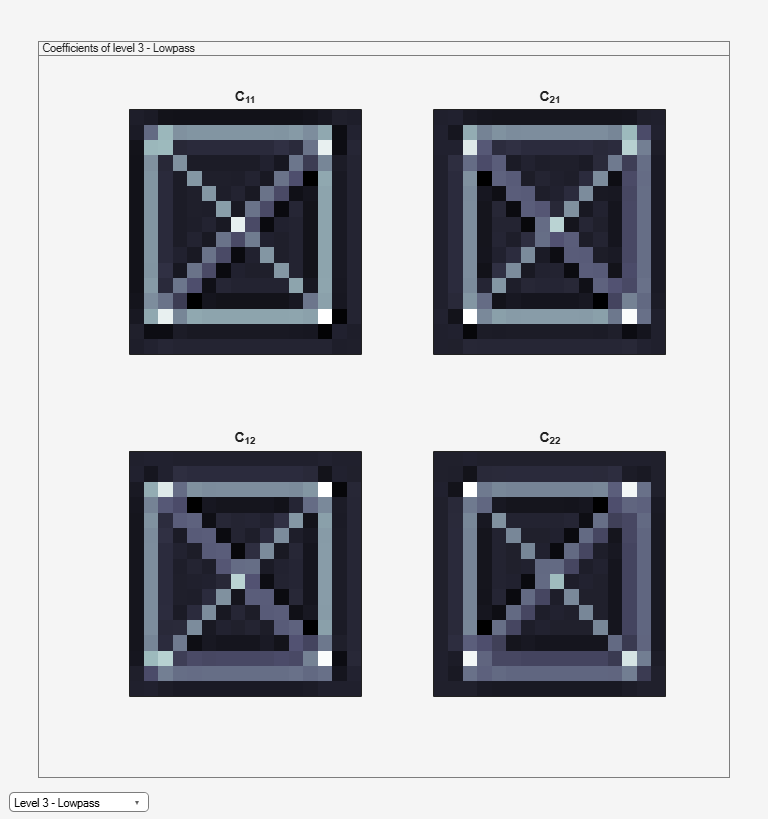# plotdt

Plot dual-tree or double-density wavelet transform

## Syntax

``plotdt(wt)``

## Description

example

````plotdt(wt)` plots the coefficients of the 1-D or 2-D wavelet filter bank decomposition, `wt`.```

## Examples

collapse all

Plot the complex dual-tree wavelet transform of the noisy Doppler signal.

Load the noisy Doppler signal. Obtain the complex dual-tree wavelet transform down to level 4.

```load noisdopp; wt = dddtree('cplxdt',noisdopp,4,'dtf1');```

Plot the coefficients.

`plotdt(wt)`Plot the complex oriented dual-tree wavelet transform of an image.

Load the `xbox` image. Obtain the complex oriented dual-tree wavelet transform down to level 3.

```load xbox; wt = dddtree2('cplxdt',xbox,3,'dtf1');```

Plot the coefficients.

`plotdt(wt)`Select the desired level detail coefficients from the drop-down list.

## Input Arguments

collapse all

Wavelet transform, returned as a structure from `dddtree` or `dddtree2` with these fields:

Type of wavelet decomposition (filter bank), specified as one of `'dwt'`, `'ddt'`, `'realdt'`, `'cplxdt'`,, `'realdddt'`, or `'cplxdddt'`. `'realdt'` and `'realdddt'` are only valid for the 2-D wavelet transform. The type, `'dwt'`, is a critically sampled (nonredundant) discrete wavelet transform for 1-D data or 2-D images. The other decomposition types are oversampled wavelet transforms. For details about transform types see `dddtree` for 1-D wavelet transforms and `dddtree2` for 2-D wavelet transforms.

Level of the wavelet decomposition, specified as a positive integer.

Decomposition (analysis) and reconstruction (synthesis) filters, specified as a structure with these fields:

First level decomposition filters specified as an N-by-2 or N-by-3 matrix for single-tree wavelet transforms, or a 1-by-2 cell array of two N-by-2 or N-by-3 matrices for dual-tree wavelet transforms. The matrices are N-by-3 for the double-density wavelet transforms. For an N-by-2 matrix, the first column of the matrix is the scaling (lowpass) filter and the second column is the wavelet (highpass) filter. For an N-by-3 matrix, the first column of the matrix is the scaling (lowpass) filter and the second and third columns are the wavelet (highpass) filters. For the dual-tree transforms, each element of the cell array contains the first-stage analysis filters for the corresponding tree.

Analysis filters for levels > 1, specified as an N-by-2 or N-by-3 matrix for single-tree wavelet transforms, or a 1-by-2 cell array of two N-by-2 or N-by-3 matrices for dual-tree wavelet transforms. The matrices are N-by-3 for the double-density wavelet transforms. For an N-by-2 matrix, the first column of the matrix is the scaling (lowpass) filter and the second column is the wavelet (highpass) filter. For an N-by-3 matrix, the first column of the matrix is the scaling (lowpass) filter and the second and third columns are the wavelet (highpass) filters. For the dual-tree transforms, each element of the cell array contains the analysis filters for the corresponding tree.

First-level reconstruction filters, specified as an N-by-2 or N-by-3 matrix for single-tree wavelet transforms, or a 1-by-2 cell array of two N-by-2 or N-by-3 matrices for dual-tree wavelet transforms. The matrices are N-by-3 for the double-density wavelet transforms. For an N-by-2 matrix, the first column of the matrix is the scaling (lowpass) filter and the second column is the wavelet (highpass) filter. For an N-by-3 matrix, the first column of the matrix is the scaling (lowpass) filter and the second and third columns are the wavelet (highpass) filters. For the dual-tree transforms, each element of the cell array contains the first-stage synthesis filters for the corresponding tree.

Reconstruction filters for levels > 1, specified as an N-by-2 or N-by-3 matrix for single-tree wavelet transforms, or a 1-by-2 cell array of two N-by-2 or N-by-3 matrices for dual-tree wavelet transforms. The matrices are N-by-3 for the double-density wavelet transforms. For an N-by-2 matrix, the first column of the matrix is the scaling (lowpass) filter and the second column is the wavelet (highpass) filter. For an N-by-3 matrix, the first column of the matrix is the scaling (lowpass) filter and the second and third columns are the wavelet (highpass) filters. For the dual-tree transforms, each element of the cell array contains the first-stage synthesis filters for the corresponding tree.

Wavelet transform coefficients, specified as a 1-by-(`level`+1) cell array of matrices. The size and structure of the matrix elements of the cell array depend on the type of wavelet transform and whether the decomposition is 1-D or 2-D. For a 1-D wavelet transform, the coefficients are organized by transform type as follows:

• `'dwt'``cfs{j}`

• j = 1,2,...`level` is the level.

• `cfs{level+1}` are the lowpass, or scaling, coefficients.

• `'ddt'``cfs{j}(:,:,k)`

• j = 1,2,... `level` is the level.

• k = 1,2 is the wavelet filter.

• `cfs{level+1}(:,:)` are the lowpass, or scaling, coefficients.

• `'cplxdt'``cfs{j}(:,:,m)`

• j = 1,2,... `level` is the level.

• m = 1,2 are the real and imaginary parts.

• `cfs{level+1}(:,:)` are the lowpass, or scaling, coefficients.

• `'realdddt'``cfs{j}(:,:,d,k)`

• j = 1,2,... `level` is the level.

• d = 1,2,3 is the orientation.

• k = 1,2 is the wavelet transform tree.

• `cfs{level+1}(:,:)` are the lowpass, or scaling, coefficients.

• `'cplxdddt'``cfs{j}(:,:,d,k,m)`

• j = 1,2,... `level` is the level.

• k = 1,2 is the wavelet transform tree.

• m = 1,2 are the real and imaginary parts.

• `cfs{level+1}(:,:)` are the lowpass, or scaling, coefficients.

For a 2-D wavelet transform, the coefficients are organized by transform type as follows:

• `'dwt'``cfs{j}(:,:,d)`

• j = 1,2,... `level` is the level.

• d = 1,2,3 is the orientation.

• `cfs{level+1}(:,:)` are the lowpass, or scaling, coefficients.

• `'ddt'``cfs{j}(:,:,d)`

• j = 1,2,... `level` is the level.

• d = 1,2,3,4,5,6,7,8 is the orientation.

• `cfs{level+1}(:,:)` are the lowpass, or scaling, coefficients.

• `'realddt'``cfs{j}(:,:,d,k)`

• j = 1,2,... `level` is the level.

• d = 1,2,3 is the orientation.

• k = 1,2 is the wavelet transform tree.

• `cfs{level+1}(:,:)` are the lowpass, or scaling, coefficients.

• `'cplxdt'``cfs{j}(:,:,d,k,m)`

• j = 1,2,... `level` is the level.

• d = 1,2,3 is the orientation.

• k = 1,2 is the wavelet transform tree.

• m = 1,2 are the real and imaginary parts.

• `cfs{level+1}(:,:)` are the lowpass, or scaling, coefficients.

• `'realdddt'``cfs{j}(:,:,d,k)`

• j = 1,2,... `level` is the level.

• d = 1,2,3 is the orientation.

• k = 1,2 is the wavelet transform tree.

• `cfs{level+1}(:,:)` are the lowpass, or scaling, coefficients.

• `'cplxdddt'``cfs{j}(:,:,d,k,m)`

• j = 1,2,... `level` is the level.

• d = 1,2,3 is the orientation.

• k = 1,2 is the wavelet transform tree.

• m = 1,2 are the real and imaginary parts.

• `cfs{level+1}(:,:)` are the lowpass, or scaling, coefficients.

## Version History

Introduced in R2013b